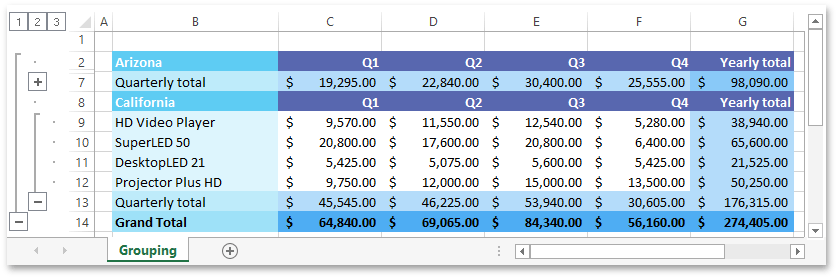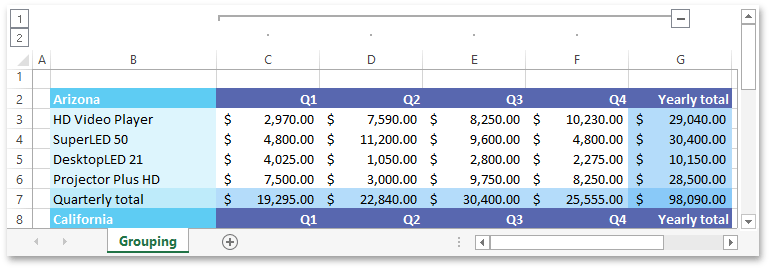21.2 (EAP/Beta)
21.1
20.2
20.1
19.2
19.1
18.2
18.1
17.2
.NET Framework 4.5.2+
.NET Framework 4.5.2+
.NET Standard 2.0+

# How to: Outline Data Manually

You can group rows and columns to make it easier to analyze a large amount of data, and simplify navigation within large worksheets. This functionality provides the capability to split your data into separate groups and hide unnecessary details in a worksheet.

Select the action you wish to perform.

## Group and Ungroup Rows

• Group

``````Worksheet worksheet = workbook.Worksheets["Grouping"];
workbook.Worksheets.ActiveWorksheet = worksheet;

// Group four rows starting from the third row and collapse the group.
worksheet.Rows.Group(2, 5, true);

// Group four rows starting from the ninth row and expand the group.
worksheet.Rows.Group(8, 11, false);

// Create the outer group of rows by grouping rows 2 through 13.
worksheet.Rows.Group(1, 12, false);
``````

The image below shows the result (the workbook is opened in Microsoft® Excel®).• Ungroup

``````Worksheet worksheet = workbook.Worksheets["Grouping and Outline"];
workbook.Worksheets.ActiveWorksheet = worksheet;

// Ungroup four rows (from the third row to the sixth row) and display collapsed data.
worksheet.Rows.UnGroup(2, 5, true);

// Ungroup four rows (from the ninth row to the twelfth row).
worksheet.Rows.UnGroup(8, 11, false);

// Remove the outer group of rows.
worksheet.Rows.UnGroup(1, 12, false);
``````

## Group and Ungroup Columns

• Group

``````Worksheet worksheet = workbook.Worksheets["Grouping"];
workbook.Worksheets.ActiveWorksheet = worksheet;

// Group four columns starting from the third column "C" and expand the group.
worksheet.Columns.Group(2, 5, false);
``````

The image below shows the result (the workbook is opened in Microsoft® Excel®).• Ungroup

``````Worksheet worksheet = workbook.Worksheets["Grouping and Outline"];
workbook.Worksheets.ActiveWorksheet = worksheet;

// Ungroup four columns (from the column "C" to the column "F").
worksheet.Columns.UnGroup(2, 5, false);
``````